# PHP Yield vs Return: Do You Know PHP Functions Can Return Values Like the Yield Statement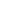PHP Classes blog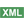PHP Yield vs Return: ...Post a comment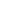See comments (0) Trackbacks (0)

Author:

Viewers: 1,368

Last month viewers: 233

Categories: PHP Tutorials

Since the concept of generator has been introduced in PHP 5.5, PHP developers can use the yield keyword in a way that looks much like a return statement, except that instead of stopping execution of the function and returning once only one value or array, yield instead generates a new value that is returned to the calling code each time it is needed.By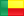<email contact>

## What Are Generators?

In case you are not aware, generators were introduced in PHP 5.5 using the yield keyword. But if you are not familiar with the concept, lets review it so you can understand why generators are useful in PHP programs.

According to Wikipedia, a generator "is very similar to a function that returns an array, in that a generator has parameters, can be called, and generates a sequence of values.

However, instead of building an array containing all the values and returning them all at once, a generator yields the values one at a time, which requires less memory and allows the caller to get started processing the first few values immediately.

In short, a generator looks like a function but behaves like an iterator".

And the PHP manual states: "A generator allows you to write code that uses foreach to iterate over a set of data without needing to build an array in memory, which could cause you to exceed a memory limit, or require a considerable amount of processing time to generate. Instead, you can write a generator function, which is the same as a normal function, except that instead of returning once, a generator can yield as many times as it needs to in order to provide the values to be iterated over.

A simple example of the utility of this feature is a new implementation of the range() function as a generator. The regular range()  function has to generate an array with every value in it and return it, which can result in large arrays: for example, calling range(0, 1000000) will result in well over 100 MB of memory being used.

As an alternative, we can implement an xrange() generator, which will only ever need enough memory to create an Iterator object and track the current state of the generator internally, which turns out to be  less than 1 kilobyte."

Let's start with this example to get back to the main point of this post "how to yield values with the return statement?":

`/* PHP doc implementation of range function using yield */function xrange( \$start, \$limit, \$step = 1) {  if (\$start < \$limit) {    if (\$step <= 0) {      throw new LogicException('Step must be +ve');    }    for (\$i = \$start; \$i <= \$limit; \$i += \$step) {      yield \$i; //use of an instance of the generator class that keep track of the state...     }  } else {    if (\$step >= 0) {      throw new LogicException('Step must be -ve');    }    for (\$i = \$start; \$i >= \$limit; \$i += \$step) {       yield \$i;//use of an instance of the generator class that keep track of the state...     }  }}`

Now as we said above we can build a basic function which has all properties of generators and then act as generator:

The properties  of such function are:

- Can been called
- Has parameters
- Keep track of actual state.

Let's try to resume this in a function yRange:

```/* Personal range function implementation without yield */function yrange( \$start, \$end, \$step=1 ) {  // 64 octets of memory usage for \$start==1
// && \$end>=10000000 and more...
static \$i=null; //keep track of state;  if(\$i===null) {    \$i=\$start;    // force Initialization;  }  if(\$start<\$end){    if(\$step <= 0) {      throw new LogicException('Step must be +ve');    }    if(\$i>\$end) {       \$i=null;      return false;    }    \$j=\$i;    \$i+=\$step;    // actual state    return \$j;    // simulate yield here;  } else {    if(\$step >= 0) {      throw new LogicException('Step must be -ve');  }  if(\$i<\$end) {   //we got an end     \$i=null;      //restart the counter    return false; //stop the loop with this...  }  \$j = \$i;  \$i += \$step;    // actual state  return \$j;      //simulate yield here;}```

and the test usage can be as follows:

```while(\$i=yrange (1,100,5)) {	echo "\$i <br>";}
foreach (xrange(1, 100, 5) as \$number) {    echo "\$number <br>";} ```
Cheers you have just built your first "generator" without using the "yield" keyword !!!

### What Did We Do Exactly?

A generator always keeps track of the actual state so we use a static variable to achieve this
and all is done.This is the only real difference between a generator and a simple function as explained above. All the logic of the implementation is detailed in comments.

### Another Example!

Aren't you are not convinced yet? Let's take another example from the PHP documentation.
```\$input = <<<'EOF'1;PHP;Likes dollar signs2;Python;Likes whitespace3;Ruby;Likes blocksEOF;/*PHP doc implementation of input_parser function using yield*/function input_parser( \$input ) {  foreach (explode("\n", \$input) as \$line) {    \$fields = explode(';', \$line);    \$id = array_shift(\$fields);    yield \$id => \$fields;  }}foreach( input_parser(\$input) as \$id => \$fields) {  echo "\$id:\n";  echo "    \$fields\n";  echo "    \$fields\n";}/* Personal input_parser function
implementation without yield */
function yInput_parser( \$input ) {  static \$i=null;                   // keep track of state;  static \$started=true;             // keep track of state;  if(empty(\$i) && \$started) {    \$i=\$input;	                    // force Initialization;  }  \$get = mb_stripos(\$i, "\n");  \$line = mb_strcut(\$i, 0, \$get);  if(!empty(\$line)) {    \$i = mb_substr(\$i, \$get + 1);               // actual state    \$line = explode(';', \$line);    return array(array_shift(\$line) => \$line);  // simulate yield here;  } else {                                      // we got an end     if(!empty(\$i)){      \$line = explode(';', \$i);      \$i = null;	                        // restart the counter      \$started=false;      return array(array_shift(\$line)=>\$line);  // simulate yield here;    }    \$i = null;    \$started = true;                            // restart the counter    return false;                   // stop the loop with this...  }}while(\$j = yinput_parser(\$input) ) {  echo key(\$j).":<br>";  echo "    ".current(\$j)."<br>";  echo "    ".current(\$j)."<br>";}```
As you may see in the comments the logic is the same as for yrange function.You can use the two functions do exactly the same thing.

### To End Definitively the Doubt about How Return can also Yield Values

Are you still in the doubt about this? Let's end definitively with the doubt with the last example:
```/* basic implementation of the PHP file function
using yield by stefan Frolich in
https://www.sitepoint.com/generators-in-php/
*/function file_lines(\$filename) {    \$file = fopen(\$filename, 'r');     while ((\$line = fgets(\$file)) !== false) {        yield \$line;     }     fclose(\$file); }foreach (file_lines(__FILE__) as \$line) {  echo '<br>'.(htmlspecialchars(\$line)).'<br>';}	/* Advanced personal implementation of the
PHP file function without yield */function yfile( \$filename, \$flags = 0, \$context = null) {  if(!file_exists( \$filename )
throw new Exception('Invalid fileName');    static \$i=null;    static \$fl=0;    static \$ct=null;    static \$key=0;    if(!is_resource( \$i )) {      \$ct = \$context;      \$fl = \$flags;      if((\$fl==1 || \$fl==7 || \$fl==5 || \$fl==3)
&& is_resource(\$ct))
\$i=fopen(\$filename, 'r',1,\$ct);      elseif((\$fl==1 || \$fl==7 || \$fl==5 || \$fl==3)
&& !is_resource(\$ct))
\$i=fopen(\$filename, 'r',1);      elseif ((\$fl!=1 && \$fl!=7 && \$fl!=5 && \$fl!=3 )
&& is_resource(\$ct))
\$i=fopen(\$filename, 'r',0,\$ct);      else \$i=fopen(\$filename, 'r');    }		      while ((\$line = fgets(\$i)) !== false) {      if(\$fl==0) {        return array(\$key++=>\$line);      }      elseif(\$fl==2 || \$fl==3) {        return array(\$key++=>rtrim(\$line));       }      elseif(\$fl==4 || \$fl==5) {        if(!ctype_space(\$line))          return array(\$key++=>\$line);	}	elseif( \$fl==6 || \$fl==7) {          if(!ctype_space(\$line))	    return array(\$key++ => rtrim(\$line));	        }					      }			      fclose(\$i);       \$i = null;      \$fl = 0;      \$ct = null;      \$key = 0;      return false;    }    \$k=0; // i just want to show 10 first lines    while (\$line =yfile( __FILE__,
FILE_USE_INCLUDE_PATH|FILE_SKIP_EMPTY_LINES)) {          echo '<br>key :'.key(\$line).':'.(htmlspecialchars(current(\$line))).'<br>';      if(\$k == 10) break;	 \$k++;      }      \$k=0; // i just want to show 10 following lines      while (\$line=yfile(__FILE__,
FILE_USE_INCLUDE_PATH|FILE_SKIP_EMPTY_LINES)) {        echo '<br>key :'.key(\$line).':'.(htmlspecialchars(current(\$line))).'<br>'; 	if(\$k == 10) break;	\$k++;      }      // i just want to show all the following lines      while (\$line = yfile(__FILE__, FILE_USE_INCLUDE_PATH|FILE_SKIP_EMPTY_LINES)) {        echo '<br>key :'.key(\$line).':'.(htmlspecialchars(current(\$line))).'<br>';      }
}
}
}```

## Keeping Track of the Internal State is the Real Secret!

As you may see the logic is again the same and it works fine...You may even built an advanced foreach loop, that keep track of where you are in the iteration even when you stopped it and restart it elsewhere in the script. So let's go for the bonus:

```function yforeach(&\$array, \$ref = true) {  static \$i=[];                          // keep track of state;  unset(\$i);                          // remove reference;  if(empty(\$i)) reset (\$array);          // bring the pointer to the start  if(list(\$i, \$i) = each(\$array)) {// actual state    if(\$ref)\$i=&\$array[\$i];        // if we want to yield reference       return \$i;                         // simulate yield here;    } else {                             // we got an end       \$i=[];                             // restart counter      return false;                      // stop the loop with this;    }  }    \$array = array(    "foo" => "bar",    "bar" => "foo",    100   => -100,    -100  => 100,  );  while(\$i = yforeach(\$array)) {            // return a reference    echo 'key:'.\$i.' value:'.\$i.'<br>';    \$i++;  }   \$array = range(1, 10);	    while(\$i = yforeach(\$array, false)) {     // without reference    echo 'key:' . \$i . ' value:' . \$i . '<br>';    \$array[\$i]++;  }
}```

Be careful !!! These implementations are not fully complete....

## Can We Send Something to the Generator just like with Generator's Send Method?

Of course a generator always keeps track of the state until it ends processing all values. So it may be useful to do send something to the generator from time to time. To achieve this PHP implements the "send" method.

Our made in house generator just act like a generator but it is not an object so what can we do? Is it really tragic? Yes of course. But don't panic. S simple trick can solve this problem elegantly.

We are creating a function and the only way to send something to a function is as an argument.
So let's take the yforeach function and implement the send method.

`function yforeach(&\$array, \$ref = true, \$send = null) {  static \$i=[];                         //keep track of state;  unset(\$i);    					  //remove reference;  if(\$send) {							  //simulate the send method here    //do something here    //just for example     if( \$send === true ) {      //do something here      \$i = array(\$send);   //actual state=send            return \$i;                         }  }                     if(empty(\$i)) reset (\$array);           // bring the pointer to the start  if(list(\$i,\$i) = each(\$array)) {  // actual state  if(\$ref)\$i = &\$array[\$i];         // if we want to yield reference     return \$i;                            // simulate yield here;  } else {                                // we got an end     \$i=[];                                // restart counter    return false;                       // stop the loop with this;  }} 	`

and the usage:

`// just for illustrate the send method \$array=range(1,10);	 \$s=0;while(\$i = yforeach(\$array, false)) {  echo 'key:'.\$i.' value:'.\$i.'<br>';  \$array[\$i]++;  if(\$s==4) {    print_r(yforeach(\$array,false,true)); //send something to the generator     break;  }  \$s++;}	while(\$i = yforeach(\$array, false)) {    echo 'key:'.\$i.' value:'.\$i.'<br>';  \$array[\$i]++;}`

## What About the wake_up Method of a Generator?

With a little imagination, you can even simulate the wake_up method using \$_SESSION array.

You can for example keep track of state in the \$_SESSION of array and resume the generator from there.

A true generator can't be serialized so this is just an example to let you know that you can simulate anything you want with a little imagination.

Not convinced of how we can do this!? Let's try it this way. Let's suppose you have a page script named 1.php with this code:

```session_start();function yforeach(&\$array, \$ref=true, \$send=null) {  static \$i=[];    					  //keep track of state;  unset(\$i);    					  //remove reference;	  if(\$send) {							  //simulate the send method here	    // do something here    // example     if( \$send === true ) {      \$i=array(\$send);          // actual state=send            return \$i;                //yield send      }  }         	                    if(empty(\$i)) reset (\$array);	       //bring the pointer to the start  if(!isset(\$_SESSION)) session_start();  if(isset(\$_SESSION['yf'])
&& isset(\$_SESSION['yf']['resume'])
&& \$_SESSION['yf']['resume'] == true) {    while(each(\$array)!=@\$_SESSION['yf']) {    }    unset(\$_SESSION['yf']);  }  if(list(\$i,\$i)=each(\$array)) {   //actual state    if(\$ref)\$i=&\$array[\$i];     //if we want to yield reference       return \$i;                        //simulate yield here;    } else {                                //we got an end       \$i=[];                            //restart counter      return false;                       //stop the loop with this;    }  }    // and the usage:  // just for illustrate the send method   \$array=range(1,10);	   \$s=0;  while(\$i=yforeach(\$array, false)) {           // without reference    echo 'key:'.\$i.' value:'.\$i.'<br>';    \$array[\$i]++;    if(\$s == 4) {      print_r(yforeach(\$array, false, true)); //send something to the generator       break;    }    \$s++;  }  \$_SESSION['yf'] = yforeach( \$array, false);
}```
and on another page 2.php this code:
```session_start();function yforeach(&\$array, \$ref=true, \$send=null) {  static \$i=[];    					  //keep track of state;  unset(\$i);    					  //remove reference;	  if(\$send) {							  //simulate the send method here    // do something here    // example     if( \$send === true ) {      \$i = array(\$send);          //actual state=send            return \$i;                //yield send      }  }         	                    if(empty(\$i)) reset (\$array);	       //bring the pointer to the start  if(!isset(\$_SESSION)) session_start();  if(isset(\$_SESSION['yf'])
&& isset(\$_SESSION['yf']['resume'])
&& \$_SESSION['yf']['resume']==true) {    while(each(\$array) != @\$_SESSION['yf']) {		    }    unset(\$_SESSION['yf']);  }  if(list(\$i,\$i) = each(\$array)) {   //actual state    if(\$ref)\$i=&\$array[\$i];     //if we want to yield reference       return \$i;                        //simulate yield here;    } else {                                //we got an end       \$i=[];                            //restart counter      return false;                       //stop the loop with this;    }  }    // and the usage:  // just for illustrate the send method
\$array=range(1,10);  \$_SESSION['yf']['resume'] = true;  \$_SESSION['keepyf'] = \$_SESSION['yf'];	  while(\$i = yforeach(\$array, false)) {         // without reference    echo 'key:'.\$i.' value:'.\$i.'<br>';    \$array[\$i]++;  }  \$_SESSION['yf']=\$_SESSION['keepyf'];  unset(\$_SESSION['keepyf']);
}```

You can try it. It is a little tricky but it can be used to simulate very closely a wake up method of a generator.

Be careful again...! The yforeach function can't replace a foreach structure because many foreach structures can be run at the same level of code but the yforeach act as a generator and then won't allow more than one instance per level and must be restart manually or automatically before use it again on a different array. If you want it act like a simple foreach you will just loose the ability to keep track of state.

As you may see you can get the same result as a true generator and even better in some cases.

Some will say: what do you do of "yield from"? My answer is yforeach is already a kind of "yield from" that you can alter to simulate the same behavior. A yield from function as yield from structure is used inside the generator.

You can for example remove the reference  on the argument \$array change each function for next and also remove the \$ref argument and the line of code which use it.

I'm sure that with a little creativity you can do something that can act as yield from.You can even simulate this behavior with a simple while or for loop without a need of

## Conclusion

Finally, even before version 5.5 of PHP you have all the necessary support to build generator or
at least a kind of generators that get the same advantages as the true generators.

In this article, you can see that with PHP, we can talk about the term "generator" as a kind of "behavior's design pattern" that we can follow in our programs to save memory usage and then increase performance.

Generator is can be a simple class or object that helps iterating on large set of data. It is above all a way to implement programming logic. And definitively "return" can also yield values!

## 1,609,176 PHP developers registered to the PHP Classes site. Be One of Us!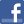Facebook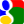Gmail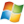Hotmail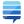StackOverflow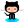GitHub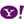YahooPHP Classes blogPHP Yield vs Return: ...Post a commentSee comments (0) Trackbacks (0)About usAdvertise on this siteSite mapNewsletterStatisticsSite tipsPrivacy policyContact
For more information send a message to `info at phpclasses dot org`.